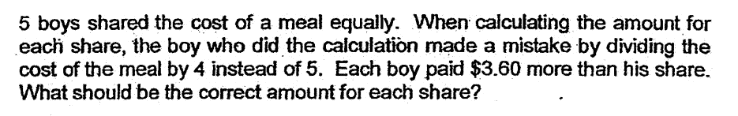# QuestionCan anybody kindly help to solve this question ?

The solution given in the answer script is \$22.50.

But my answer is \$14.40, maybe I mis-understood the question ?

Thank you in advanced.

1 Answer

# Answer

\$14.4 is correct.

Let x be the cost of meal

x/4 – x/5 = 3.6

x = 72

72/5 = 14.4

0 Replies 0 Likes ✔Accepted Answer